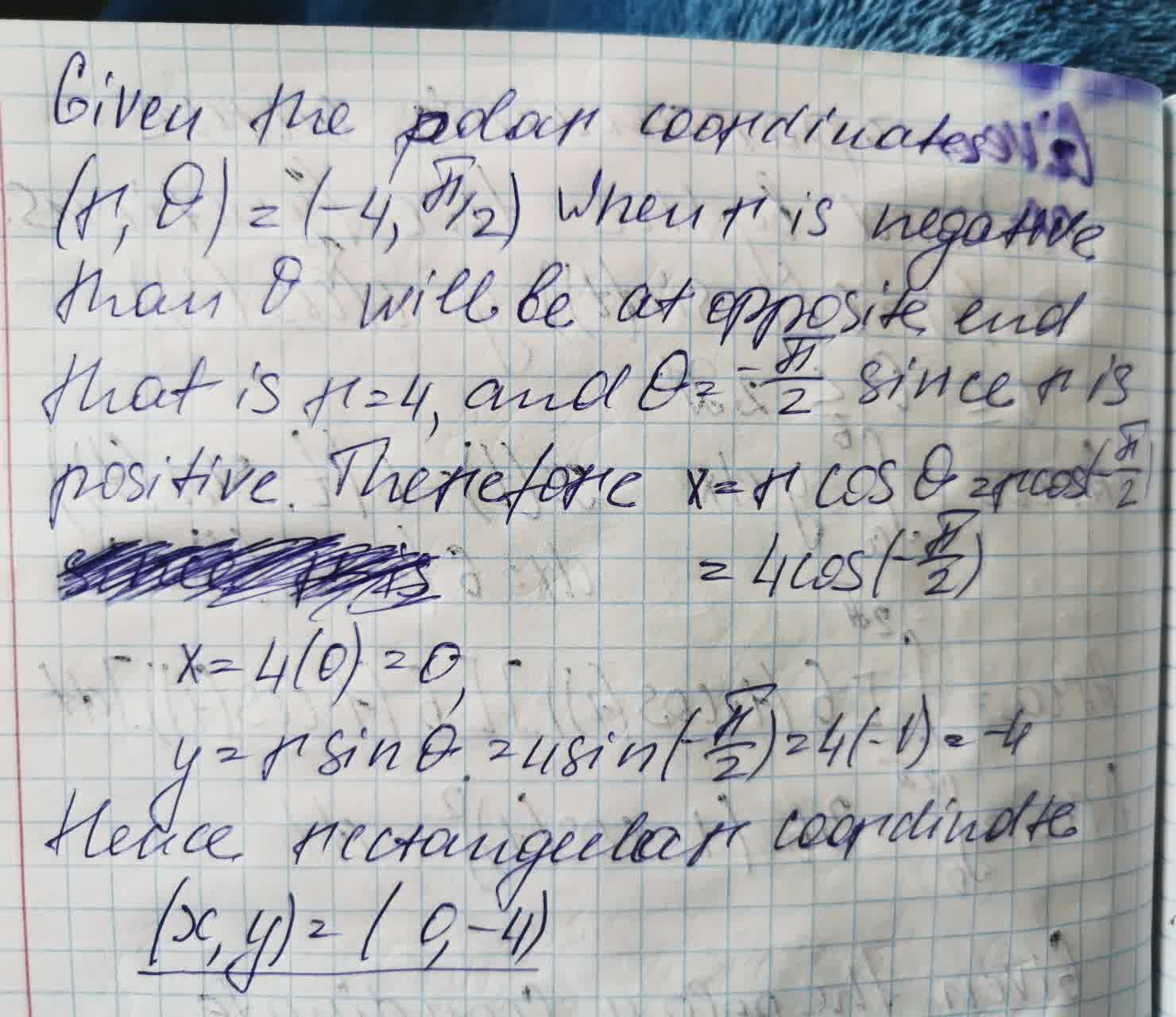# The quadratic formula is used to solve for x in equations taking the form of a quadratic equation, ax^{2}+bc+c=0 solve for x in the expression using the quadratic formula. 2x^{2}+15x−9.1=0Ava-May Nelson 2021-09-02 Answered
The quadratic formula is used to solve for x in equations taking the form of a quadratic equation, $$\displaystyle{a}{x}^{{{2}}}+{b}{c}+{c}={0}$$
solve for x in the expression using the quadratic formula.
$$\displaystyle{2}{x}^{{{2}}}+{15}{x}−{9.1}={0}$$

• Questions are typically answered in as fast as 30 minutes

### Plainmath recommends

• Get a detailed answer even on the hardest topics.
• Ask an expert for a step-by-step guidance to learn to do it yourself.Szeteib

Solution below